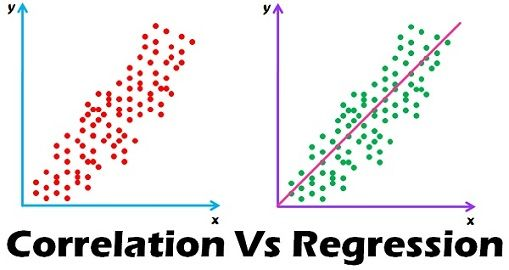#Asim Jana
• Male
• Kolkata, West Bengal
• India# Asim Jana's Page

## Latest Activity"Actually Alok, Asim is correct in his article. The correlation coefficient of your x and y is .975, I got the same result whether calculated by hand using the Pearson formula or calculated using R's cor(). Just because each y is a multiple or…"
Mar 28, 2018"Hi Alok, Very effective comment. See my below comments. Two variables are said to be "correlated" or "associated" if knowing scores for one of them helps to predict scores for the other.  Capacity to predict is measured by a…"
Mar 27, 2018""Correlation coefficient indicates the extent to which two variables move together." - not really. Illustration - x (1,2,3,4,5,6,7,8, 9) and y (1,4,9,16,25,36,49,64,81) -  x and y here move together. But what is the correlation…"
Mar 21, 2018Asim Jana posted a blog post

### Difference Between Correlation and Regression in Statistics

Correlation and Regression are the two analysis based on multivariate distribution. A multivariate distribution is described as a distribution of multiple variables. Correlation is described as the analysis which lets us know the association or the absence of the relationship between two variables ‘x’ and ‘y’. On the other end, Regression analysis, predicts the value of the dependent variable…See More
Mar 9, 2018Asim Jana's blog post was featured

### Difference Between Correlation and Regression in Statistics

Correlation and Regression are the two analysis based on multivariate distribution. A multivariate distribution is described as a distribution of multiple variables. Correlation is described as the analysis which lets us know the association or the absence of the relationship between two variables ‘x’ and ‘y’. On the other end, Regression analysis, predicts the value of the dependent variable…See More
Mar 9, 2018

## Profile Information

Short Bio
Data Scientist
My Web Site Or LinkedIn Profile
Professional Status
Technical
Years of Experience:
11
IBM
Industry:
IT
Data Scientist
How did you find out about DataScienceCentral?
Other
Interests:
Contributing, Networking, Finding a new position, New venture, Other
What is your Favorite Data Mining or Analytical Website?
http://https://www.analyticsvidhya.com/

## Asim Jana's Blog

### Difference Between Correlation and Regression in Statistics

Posted on March 8, 2018 at 3:30pmCorrelation and Regression are the two analysis based on multivariate distribution. A multivariate distribution is described as a distribution of multiple variables. Correlation is described as the analysis which lets us know the association or the absence of the relationship between two variables ‘x’ and ‘y’. On the other end, Regression…

Continue

## Comment Wall

Join Data Science Central